Publicité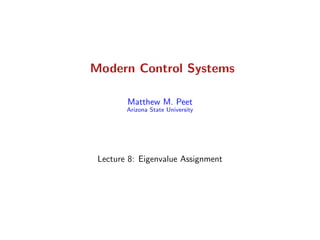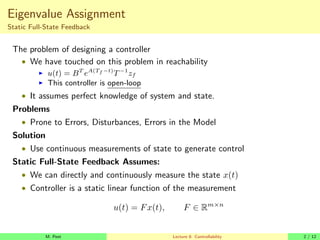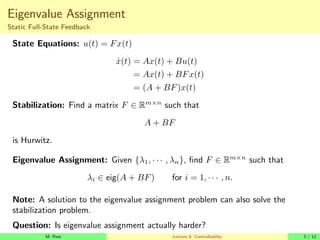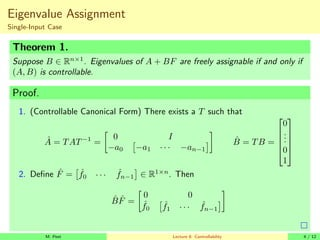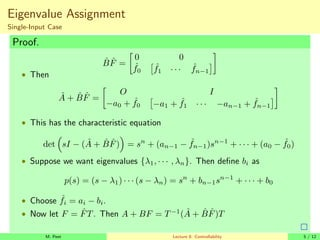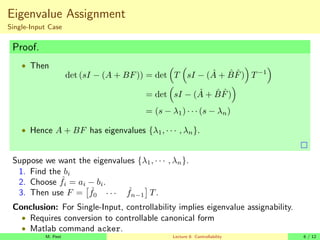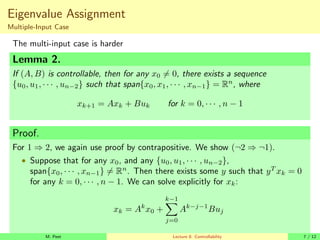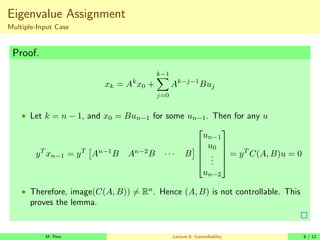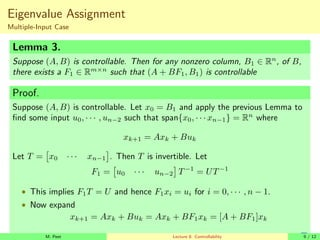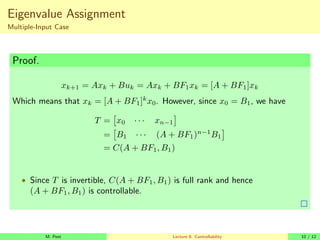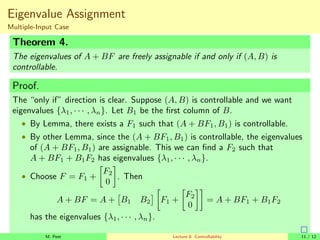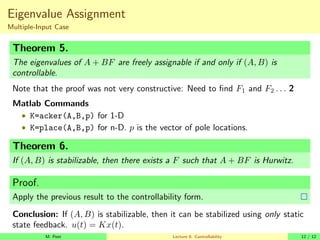1 sur 12
Publicité

### 507Lecture08.pdf

1. Modern Control Systems Matthew M. Peet Arizona State University Lecture 8: Eigenvalue Assignment
2. Eigenvalue Assignment Static Full-State Feedback The problem of designing a controller • We have touched on this problem in reachability I u(t) = BT eA(Tf −t) T−1 zf I This controller is open-loop • It assumes perfect knowledge of system and state. Problems • Prone to Errors, Disturbances, Errors in the Model Solution • Use continuous measurements of state to generate control Static Full-State Feedback Assumes: • We can directly and continuously measure the state x(t) • Controller is a static linear function of the measurement u(t) = Fx(t), F ∈ Rm×n M. Peet Lecture 8: Controllability 2 / 12
3. Eigenvalue Assignment Static Full-State Feedback State Equations: u(t) = Fx(t) ẋ(t) = Ax(t) + Bu(t) = Ax(t) + BFx(t) = (A + BF)x(t) Stabilization: Find a matrix F ∈ Rm×n such that A + BF is Hurwitz. Eigenvalue Assignment: Given {λ1, · · · , λn}, find F ∈ Rm×n such that λi ∈ eig(A + BF) for i = 1, · · · , n. Note: A solution to the eigenvalue assignment problem can also solve the stabilization problem. Question: Is eigenvalue assignment actually harder? M. Peet Lecture 8: Controllability 3 / 12
4. Eigenvalue Assignment Single-Input Case Theorem 1. Suppose B ∈ Rn×1 . Eigenvalues of A + BF are freely assignable if and only if (A, B) is controllable. Proof. 1. (Controllable Canonical Form) There exists a T such that Â = TAT−1 = 0 I −a0 −a1 · · · −an−1 B̂ = TB =      0 . . . 0 1      2. Define F̂ = ˆ f0 · · · ˆ fn−1 ∈ R1×n . Then B̂F̂ = 0 0 ˆ f0 ˆ f1 · · · ˆ fn−1 M. Peet Lecture 8: Controllability 4 / 12
5. Eigenvalue Assignment Single-Input Case Proof. B̂F̂ = 0 0 ˆ f0 ˆ f1 · · · ˆ fn−1 • Then Â + B̂F̂ = O I −a0 + ˆ f0 −a1 + ˆ f1 · · · −an−1 + ˆ fn−1 • This has the characteristic equation det sI − (Â + B̂F̂) = sn + (an−1 − ˆ fn−1)sn−1 + · · · + (a0 − ˆ f0) • Suppose we want eigenvalues {λ1, · · · , λn}. Then define bi as p(s) = (s − λ1) · · · (s − λn) = sn + bn−1sn−1 + · · · + b0 • Choose ˆ fi = ai − bi. • Now let F = F̂T. Then A + BF = T−1 (Â + B̂F̂)T M. Peet Lecture 8: Controllability 5 / 12
6. Eigenvalue Assignment Single-Input Case Proof. • Then det (sI − (A + BF)) = det T sI − (Â + B̂F̂) T−1 = det sI − (Â + B̂F̂) = (s − λ1) · · · (s − λn) • Hence A + BF has eigenvalues {λ1, · · · , λn}. Suppose we want the eigenvalues {λ1, · · · , λn}. 1. Find the bi 2. Choose ˆ fi = ai − bi. 3. Then use F = ˆ f0 · · · ˆ fn−1 T. Conclusion: For Single-Input, controllability implies eigenvalue assignability. • Requires conversion to controllable canonical form • Matlab command acker. M. Peet Lecture 8: Controllability 6 / 12
7. Eigenvalue Assignment Multiple-Input Case The multi-input case is harder Lemma 2. If (A, B) is controllable, then for any x0 6= 0, there exists a sequence {u0, u1, · · · , un−2} such that span{x0, x1, · · · , xn−1} = Rn , where xk+1 = Axk + Buk for k = 0, · · · , n − 1 Proof. For 1 ⇒ 2, we again use proof by contrapositive. We show (¬2 ⇒ ¬1). • Suppose that for any x0, and any {u0, u1, · · · , un−2}, span{x0, · · · , xn−1} 6= Rn . Then there exists some y such that yT xk = 0 for any k = 0, · · · , n − 1. We can solve explicitly for xk: xk = Ak x0 + k−1 X j=0 Ak−j−1 Buj M. Peet Lecture 8: Controllability 7 / 12
8. Eigenvalue Assignment Multiple-Input Case Proof. xk = Ak x0 + k−1 X j=0 Ak−j−1 Buj • Let k = n − 1, and x0 = Bun−1 for some un−1. Then for any u yT xn−1 = yT An−1 B An−2 B · · · B      un−1 u0 . . . un−2      = yT C(A, B)u = 0 • Therefore, image(C(A, B)) 6= Rn . Hence (A, B) is not controllable. This proves the lemma. M. Peet Lecture 8: Controllability 8 / 12
9. Eigenvalue Assignment Multiple-Input Case Lemma 3. Suppose (A, B) is controllable. Then for any nonzero column, B1 ∈ Rn , of B, there exists a F1 ∈ Rm×n such that (A + BF1, B1) is controllable Proof. Suppose (A, B) is controllable. Let x0 = B1 and apply the previous Lemma to find some input u0, · · · , un−2 such that span{x0, · · · xn−1} = Rn where xk+1 = Axk + Buk Let T = x0 · · · xn−1 . Then T is invertible. Let F1 = u0 · · · un−2 T−1 = UT−1 • This implies F1T = U and hence F1xi = ui for i = 0, · · · , n − 1. • Now expand xk+1 = Axk + Buk = Axk + BF1xk = [A + BF1]xk M. Peet Lecture 8: Controllability 9 / 12
10. Eigenvalue Assignment Multiple-Input Case Proof. xk+1 = Axk + Buk = Axk + BF1xk = [A + BF1]xk Which means that xk = [A + BF1]k x0. However, since x0 = B1, we have T = x0 · · · xn−1 = B1 · · · (A + BF1)n−1 B1 = C(A + BF1, B1) • Since T is invertible, C(A + BF1, B1) is full rank and hence (A + BF1, B1) is controllable. M. Peet Lecture 8: Controllability 10 / 12
11. Eigenvalue Assignment Multiple-Input Case Theorem 4. The eigenvalues of A + BF are freely assignable if and only if (A, B) is controllable. Proof. The “only if” direction is clear. Suppose (A, B) is controllable and we want eigenvalues {λ1, · · · , λn}. Let B1 be the first column of B. • By Lemma, there exists a F1 such that (A + BF1, B1) is controllable. • By other Lemma, since the (A + BF1, B1) is controllable, the eigenvalues of (A + BF1, B1) are assignable. This we can find a F2 such that A + BF1 + B1F2 has eigenvalues {λ1, · · · , λn}. • Choose F = F1 + F2 0 . Then A + BF = A + B1 B2 F1 + F2 0 = A + BF1 + B1F2 has the eigenvalues {λ1, · · · , λn}. M. Peet Lecture 8: Controllability 11 / 12
12. Eigenvalue Assignment Multiple-Input Case Theorem 5. The eigenvalues of A + BF are freely assignable if and only if (A, B) is controllable. Note that the proof was not very constructive: Need to find F1 and F2 . . . 2 Matlab Commands • K=acker(A,B,p) for 1-D • K=place(A,B,p) for n-D. p is the vector of pole locations. Theorem 6. If (A, B) is stabilizable, then there exists a F such that A + BF is Hurwitz. Proof. Apply the previous result to the controllability form. Conclusion: If (A, B) is stabilizable, then it can be stabilized using only static state feedback. u(t) = Kx(t). M. Peet Lecture 8: Controllability 12 / 12
Publicité